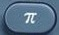# πBasics

π = pi

π = approximately 3.141592654 = approximately 3.14
π = ratio of a circle’s circumference to its diameter
π is primarily a multiplier.

When π is immediately adjacent to a number, letter or parenthesis, an invisible multiplication sign is in force.
5π = 5 × π
π5 = π × 5
rπ = r × π
πr = π × r
π(5) = π × 5
5(π) = 5 × π
π(r) = π × r
r(π) = r × π

When asked to convert an answer from π to decimal form, use the toggle button.is the π command.

Question

What is 5 × π?

Input Display Commentblinker clears screen
5 ×5*πAnswer
###### π

Question

What is π × 5?

Input Display Commentblinker clears screen× 5 π*5Answer
###### π

Question

In decimal form, what is 5 × π?

15.70796327

See Toggle.
5π ⇔ 15.70796327

Input Display Commentblinker clears screen
5 ×5*π15.70796327 Answer
(Toggle)
###### π

Question

With reference to the question above, round your answer to the nearest hundredth.

15.71

See Rounding.
15.70796327  15.71

Practice – Questions
1.  What is 2 × π?

2.  What is π × 2?

3.  In decimal form, what is 2 × π?

4.  With reference to Question 3, round your answer to the nearest hundredth.

5.  With reference to Question 3, round your answer to the nearest tenth.

6.  What is 8 × π?

7.  What is π × 8?

8.  In decimal form, what is 8 × π?

9.  With reference to Question 8, round your answer to the nearest hundredth.

10.  With reference to Question 8, round your answer to the nearest tenth.

1.  2π

2.  2π

3.  6.283185307

4.  6.28

5.  6.3

6.  8π

7.  8π

8.  25.13274123

9.  25.13

10.  25.1